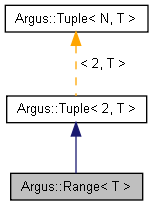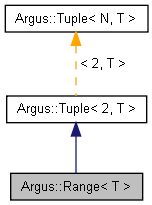## Jetson Linux Multimedia API Reference

#### 32.4.3 Release

Argus::Range< T > Class Template Reference

## Detailed Description

### template<typename T> class Argus::Range< T >

Range template class.

This is a Tuple specialization containing 2 elements corresponding to the min and max values of the range, in that order. Values can be accessed using the named methods.

Definition at line 718 of file Types.h.

Inheritance diagram for Argus::Range< T >:[legend]
Collaboration diagram for Argus::Range< T >:[legend]

## Public Member Functions

Range ()

Range (const Tuple< 2, T > &other)

Range (T init)

Range (T _min, T _max)

T & min ()

const T & min () const

T & max ()

const T & max () const

bool empty () const

bool operator== (const Tuple< N, T > &rhs) const
Returns true when every element in the two tuples are identical. More...

bool operator!= (const Tuple< N, T > &rhs) const
Returns true if there are any differences between the two tuples. More...

Tuple< N, T > & operator+= (const Tuple< N, T > &rhs)
Adds every element of another tuple to the elements of this tuple. More...

Tuple< N, T > & operator-= (const Tuple< N, T > &rhs)
Subtracts every element of another tuple from the elements of this tuple. More...

Tuple< N, T > & operator*= (const T &rhs)
Multiplies every element in the tuple by a single value. More...

Tuple< N, T > & operator/= (const T &rhs)
Divides every element in the tuple by a single value. More...

const Tuple< N, T > operator+ (const Tuple< N, T > &rhs) const
Returns the result of adding another tuple to this tuple. More...

const Tuple< N, T > operator- (const Tuple< N, T > &rhs) const
Returns the result of subtracting another tuple from this tuple. More...

const Tuple< N, T > operator* (const T &rhs) const
Returns the result of multiplying this tuple by a single value. More...

const Tuple< N, T > operator/ (const T &rhs) const
Returns the result of dividing this tuple by a single value. More...

T & operator[] (unsigned int i)

const T & operator[] (unsigned int i) const

## Static Public Member Functions

static unsigned int tupleSize ()
Returns the number of elements in the tuple. More...

m_data [N]

## Constructor & Destructor Documentation

template<typename T>
 Argus::Range< T >::Range ( )
inline

Definition at line 721 of file Types.h.

template<typename T>
 Argus::Range< T >::Range ( const Tuple< 2, T > & other )
inline

Definition at line 722 of file Types.h.

template<typename T>
 Argus::Range< T >::Range ( T init )
inline

Definition at line 724 of file Types.h.

References Argus::Range< T >::max(), and Argus::Range< T >::min().

template<typename T>
 Argus::Range< T >::Range ( T _min, T _max )
inline

Definition at line 729 of file Types.h.

References Argus::Range< T >::max(), and Argus::Range< T >::min().

## Member Function Documentation

template<typename T>
 bool Argus::Range< T >::empty ( ) const
inline

Definition at line 740 of file Types.h.

References Argus::Range< T >::max(), and Argus::Range< T >::min().

template<typename T>
 T& Argus::Range< T >::max ( )
inline

Definition at line 737 of file Types.h.

Referenced by Argus::Range< T >::empty(), and Argus::Range< T >::Range().

template<typename T>
 const T& Argus::Range< T >::max ( ) const
inline

Definition at line 738 of file Types.h.

template<typename T>
 T& Argus::Range< T >::min ( )
inline

Definition at line 735 of file Types.h.

Referenced by Argus::Range< T >::empty(), and Argus::Range< T >::Range().

template<typename T>
 const T& Argus::Range< T >::min ( ) const
inline

Definition at line 736 of file Types.h.

 bool Argus::Tuple< N, T >::operator!= ( const Tuple< N, T > & rhs ) const
inlineinherited

Returns true if there are any differences between the two tuples.

Definition at line 471 of file Types.h.

 const Tuple Argus::Tuple< N, T >::operator* ( const T & rhs ) const
inlineinherited

Returns the result of multiplying this tuple by a single value.

Definition at line 521 of file Types.h.

 Tuple& Argus::Tuple< N, T >::operator*= ( const T & rhs )
inlineinherited

Multiplies every element in the tuple by a single value.

Definition at line 493 of file Types.h.

References Argus::Tuple< N, T >::m_data.

 const Tuple Argus::Tuple< N, T >::operator+ ( const Tuple< N, T > & rhs ) const
inlineinherited

Returns the result of adding another tuple to this tuple.

Definition at line 509 of file Types.h.

 Tuple& Argus::Tuple< N, T >::operator+= ( const Tuple< N, T > & rhs )
inlineinherited

Adds every element of another tuple to the elements of this tuple.

Definition at line 477 of file Types.h.

References Argus::Tuple< N, T >::m_data.

 const Tuple Argus::Tuple< N, T >::operator- ( const Tuple< N, T > & rhs ) const
inlineinherited

Returns the result of subtracting another tuple from this tuple.

Definition at line 515 of file Types.h.

 Tuple& Argus::Tuple< N, T >::operator-= ( const Tuple< N, T > & rhs )
inlineinherited

Subtracts every element of another tuple from the elements of this tuple.

Definition at line 485 of file Types.h.

References Argus::Tuple< N, T >::m_data.

 const Tuple Argus::Tuple< N, T >::operator/ ( const T & rhs ) const
inlineinherited

Returns the result of dividing this tuple by a single value.

Definition at line 527 of file Types.h.

 Tuple& Argus::Tuple< N, T >::operator/= ( const T & rhs )
inlineinherited

Divides every element in the tuple by a single value.

Definition at line 501 of file Types.h.

References Argus::Tuple< N, T >::m_data.

 bool Argus::Tuple< N, T >::operator== ( const Tuple< N, T > & rhs ) const
inlineinherited

Returns true when every element in the two tuples are identical.

Definition at line 465 of file Types.h.

References Argus::Tuple< N, T >::m_data.

 T& Argus::Tuple< N, T >::operator[] ( unsigned int i )
inlineinherited

Definition at line 532 of file Types.h.

References Argus::Tuple< N, T >::m_data.

 const T& Argus::Tuple< N, T >::operator[] ( unsigned int i ) const
inlineinherited

Definition at line 533 of file Types.h.

References Argus::Tuple< N, T >::m_data.

 static unsigned int Argus::Tuple< N, T >::tupleSize ( )
inlinestaticinherited

Returns the number of elements in the tuple.

Definition at line 536 of file Types.h.

## Field Documentation

 T Argus::Tuple< N, T >::m_data[N]
protectedinherited

Definition at line 539 of file Types.h.

The documentation for this class was generated from the following file: